### Perimeter and Area - Worksheets

CBSE Worksheet-01

Class - IV Mathematics – Perimeter and Area

Time Allowed: 30 minutes

1. Fill in the blanks with suitable words:
1. Area of a square is given by _______.

2. Perimeter of a rectangle = 2 $×$ (length + _______).

3. The two sides of a triangle are 3 cm and 4 cm and its perimeter is 15 cm.The third side is _______ cm.

4. One side of a square whose perimeter is 4 cm is ______.

5. The perimeter of the square whose side is 20 cm is ______.

2. State whether each of the following statement is True or False:
1. Perimeter can be measured in units i.e. cm, m, km, etc.

2. Area of square of one side 5 cm is 25 cm2

3. Perimeter of square = side $×$ side

4. Area of a rectangle of length 12 cm and breadth 4 cm = 48 m

5. The multiplication of all the length of figure is called it's perimeter.

3. Pritty wants to put a new cabinet in one side of her kitchen. She measures one side of her kitchen and finds it measures 18 meter. Which measurement did Pritty find?

1. Length

3. Perimeter

4. Area

4. Area of square is

1. $×$ Side

2. Side $×$ Side

3. Side + Side

4. $×$ Side

5. Which of the following formulas, or rules, cannot be used to calculate the perimeter of a rectangle?

1. Perimeter = length + width + length + width

2. Perimeter = length + width

3. Perimeter = 2(length + width)

4. Perimeter = 2 length + 2 width

6. The perimeter of the rectangle is 90 cm. The length is 27 cm. What is the breadth of the rectangle?

1. 20 cm

2. 18 cm

3. 21 cm

4. 19 cm

7. Which of the following statement describes the term 'Perimeter' correctly:

1. The multiplication of all the lengths of a figure is called its perimeter.

2. None of these.

3. Perimeter can be defined as the amount of surface covered by any figure or object.

4. The sum of all the lengths of a figure is called its perimeter.

8. Match the following:

 (a)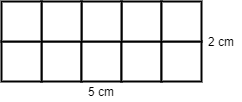(i) Perimeter = 12 cm (b)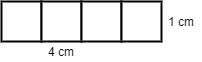(ii) Perimeter = 16 cm (c)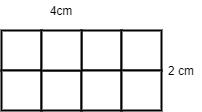(iii) Perimeter = 14 cm (d)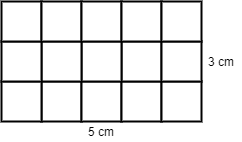(iv) Perimeter = 10 cm
9. If the cost of fencing is Rs 25 per meter. How much does it cost to fence the given below field?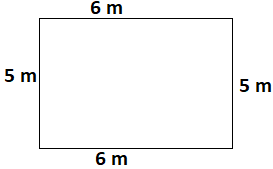10. Raheem bought some wrapping paper which was 9 ft. long and 9 ft. wide. What is the perimeter of the wrapping paper he bought?

11.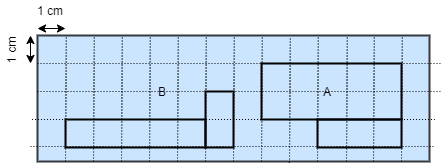Each figure is made up of two rectangles. Find the area and Perimeter of the above figure.

12. Arav is a nameplate designer. He designed a nameplate that was 10 inches by 15 inches. The cost to design the quality plate is Rs13 per square inch. What was the total cost of the nameplate?

13.  Rachel was cutting out some fabric for a friend. She cut a piece that was 5 centimeters wide and had an area of 20 cm2. How long was the piece?

14. Two square tiles, each with a side of 3 cm, are placed side by side to form a rectangle. What is the area of the rectangle?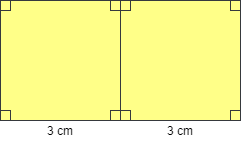15. The figure is made up of two rectangles. Find its area.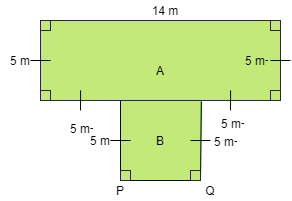16. Find the perimeter of the given figures:

1.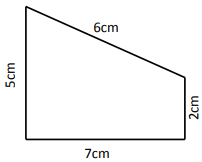2.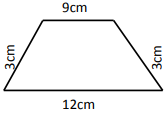3.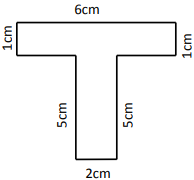4.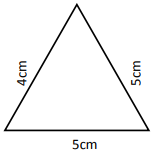CBSE Worksheet-01
Class - IV Mathematics – Perimeter and Area

Solution

1. Fill in the blanks with suitable words:

1. Side2 or side $×$ side.

2. Breadth. Perimeter of a rectangle = 2$×$(length + breadth).

3. 8 cm.
Perimeter of triangle = sum of all sides.
So, Perimeter of triangle = 3 + 4 + 3rd side
15 = 3 + 4 + third side ... (perimeter is 15 cm given)
third side = 15 - 7
Therefore, third side = 8 cm.

4. 1 cm.
Perimeter of square = 4 $×$ side.
So, Perimeter of square = 4 $×$ side
4 = 4 $×$ side ... (perimeter = 4 cm given)
side = $\frac{4}{4}$
Therefore, side = 1 cm.

5. The perimeter of the square whose side is 20 cm is 80 cm. [ P = 4 side = 4 $×$ 20 = 80 ]

2. State whether each of the following statement is True or False:

1. True

2. True, Area of square = side $×$ side
= 5$×$5 sq. cm
= 25 cm2

3. False, perimeter of square = 4 $×$ side

4. False, area of the rectangle with length 12 cm and breadth 4 cm is 48 cm2

5. False, the addition of all the length of a closed figure is called it's perimeter.

3. (a) Length
Explanation: Pritty wants to put cabinets in only one side of kitchen, so she wants to measure the length of only one side
4. (b) Side $×$ Side
Explanation: Area of Square = Side $×$ Side
5. (b) Perimeter = length + width
Explanation: Perimeter = length + width. This expression is incorrect because perimeter = 2 $×$ (length + breadth/ width) and we can it as Perimeter = length + width + length + width or Perimeter = 2 length + 2 width.
6. (b) 18 cm
Explanation: Perimeter of rectangle = 2 $×$ length + 2 $×$ breadth
90 = 2 $×$ 27 + 2 $×$ breadth
90 = 54 + 2 $×$ breadth
$×$ breadth = 90 - 54
$×$ breadth = 36
Breadth = 36 $÷$ 2
7. (d) The sum of all the length of a figure is called its perimeter.
Explanation: The sum of all the length of a figure is called its perimeter.
8. Formula used is sum of all sides= Perimeter.
(a)-(iii), (b)-(iv), (c)-(i), (d)-(ii)

9. To find the cost of fencing first we need to find the perimeter of field:
then, perimeter of field = 2 $×$ (length + breadth)
= 2 $×$ (6 + 5) = 2 $×$ 11 = 22 m
now, the cost of fencing = 22 $×$ 25 = Rs 550

10. Length of wrapping paper = 9 ft.
Breadth of wrapping paper 9 ft.
Perimeter of the wrapping paper = 4 $×$ side
= 4 $×$ 9 ft.
= 36 ft.

11. Area of figure A = 10 + 3 = 13 cm2
Perimeter of figure A = 16 cm
Area of figure B =5 + 2 = 7 cm2
Perimeter of figure B = 16 cm

12. Length of name plate = 10 inches
Breadth of nameplate = 15 inches
Area of name plate = Length $×$ Breadth
= 10 $×$ 15 sq. inches
= 150 sq. inches
ATQ
The cost to design the quality plate is Rs. 13 per square inch.
The total cost of the nameplate = Total area $×$ Rate
= 150 $×$ 13
= Rs. 1950
Hence the total cost of the nameplate is Rs. 1950

13.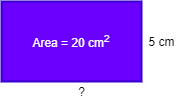ATQ
Area of piece of fabric = Length $×$ Breadth
20 = Length $×$ 5
Length = 20 $÷$ 5
= 4 cm
Hence the length of piece of fabric is 4 cm

14.Length of rectangle = 3 + 3 = 6 cm
breadth of rectangle = 3 cm
Area of rectangle = 6 $×$ 3
= 18 cm2

15.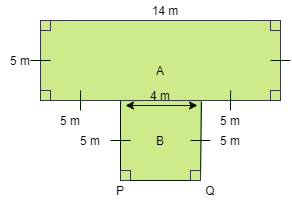Area of figure = Area of rectangle A + area of rectangle B
Length of rectangle A = 14 m
Breadth of rectangle A = 5 m
Area of rectangle A = 14 $×$ 5
= 60 m2
Length of rectangle B = 14-(5+5) m = 14 - 10 = 4 m
Breadth of rectangle A = 5 m
Area of rectangle B = 4 $×$ 5
= 20 m2
Area of figure = 60+20 m2
=80 m2
Hence the area of figure is 80 m2

1.Perimeter of figure =  sum of all sides
= 5 + 6 + 2 + 7 = 20 cm
2.Perimeter of figure =  sum of all sides
= 3 + 9 + 3 + 12 = 27 cm
3.Perimeter of figure =  sum of all sides
= 2 + 5 + 1 + 6 + 1 + 5 = 20 cm
4.Perimeter of figure =  sum of all sides
= 5 + 4 + 5 = 14 cm